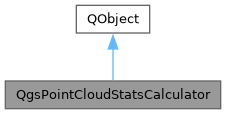QGIS API Documentation 3.27.0-Master (a46f227e17)
QgsPointCloudStatsCalculator Class Reference

Class used to calculate statistics of a point cloud dataset. More...

`#include <qgspointcloudstatscalculator.h>`

Inheritance diagram for QgsPointCloudStatsCalculator:[legend]

## Public Member Functions

QgsPointCloudStatsCalculator (QgsPointCloudIndex *index)
Constructor. More...

bool calculateStats (QgsFeedback *feedback, const QVector< QgsPointCloudAttribute > &attributes, qint64 pointsLimit=-1)
Calculates the statistics of given attributes attributes up to new pointsLimit points Note: the already calculated statistics are kept and another set of pointsLimit are processed. More...

QgsPointCloudStatistics statistics () const
Returns the object containing the calculated statistics. More...

## Detailed Description

Class used to calculate statistics of a point cloud dataset.

Since
QGIS 3.26

Definition at line 49 of file qgspointcloudstatscalculator.h.

## ◆ QgsPointCloudStatsCalculator()

 QgsPointCloudStatsCalculator::QgsPointCloudStatsCalculator ( QgsPointCloudIndex * index )

Constructor.

Definition at line 172 of file qgspointcloudstatscalculator.cpp.

## ◆ calculateStats()

 bool QgsPointCloudStatsCalculator::calculateStats ( QgsFeedback * feedback, const QVector< QgsPointCloudAttribute > & attributes, qint64 pointsLimit = `-1` )

Calculates the statistics of given attributes attributes up to new pointsLimit points Note: the already calculated statistics are kept and another set of pointsLimit are processed.

Definition at line 178 of file qgspointcloudstatscalculator.cpp.

## ◆ statistics()

 QgsPointCloudStatistics QgsPointCloudStatsCalculator::statistics ( ) const
inline

Returns the object containing the calculated statistics.

Definition at line 63 of file qgspointcloudstatscalculator.h.

The documentation for this class was generated from the following files: